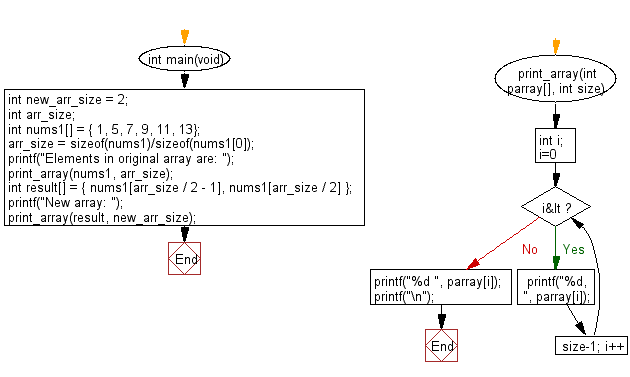﻿ C Program: Array of even-length with 2 middle elements# C Exercises: Create an array taking two middle elements from a given array of integers of length even

## C-programming basic algorithm: Exercise-46 with Solution

Write a C program to create an array taking two middle elements from a given array of integers of length even.

C Code:

``````#include <stdio.h>
#include <stdlib.h>
int main(void){
int new_arr_size = 2;
int arr_size;
int nums1[] = { 1, 5, 7, 9, 11, 13};
arr_size = sizeof(nums1)/sizeof(nums1);
printf("Elements in original array are: ");
print_array(nums1, arr_size);
int result[] = { nums1[arr_size / 2 - 1], nums1[arr_size / 2] };
printf("New array: ");
print_array(result, new_arr_size);
}
print_array(int parray[], int size)
{
int i;
for( i=0; i<size-1; i++)
{
printf("%d, ", parray[i]);
}
printf("%d ", parray[i]);
printf("\n");
}
``````

Sample Output:

```Elements in original array are: 1, 5, 7, 9, 11, 13
New array: 7, 9
```

Pictorial Presentation:Flowchart:C Programming Code Editor:

What is the difficulty level of this exercise?

Test your Programming skills with w3resource's quiz.

﻿

## C Programming: Tips of the Day

Why doesn't a+++++b work?

printf("%d",a+++++b); is interpreted as (a++)++ + b according to the Maximal Munch Rule!.

++ (postfix) doesn't evaluate to an lvalue but it requires its operand to be an lvalue.

! 6.4/4 says the next preprocessing token is the longest sequence of characters that could constitute a preprocessing token"

Ref : https://bit.ly/3fdldUT